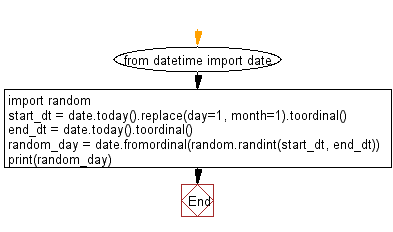﻿ Python Math: Select a random date in the current year - w3resource# Python Math: Select a random date in the current year

## Python Math: Exercise-74 with Solution

Write a Python program to select a random date in the current year.

Sample Solution:-

Python Code:

``````from datetime import date
import random

start_dt = date.today().replace(day=1, month=1).toordinal()
end_dt = date.today().toordinal()
random_day = date.fromordinal(random.randint(start_dt, end_dt))
print(random_day)
```
```

Sample Output:

```2017-02-19
```

Flowchart:## Visualize Python code execution:

The following tool visualize what the computer is doing step-by-step as it executes the said program:

Python Code Editor:

Have another way to solve this solution? Contribute your code (and comments) through Disqus.

What is the difficulty level of this exercise?

Test your Programming skills with w3resource's quiz.

﻿

```>>> students = [{'name': 'John', 'score': 98}, {'name': 'Mike', 'score': 94}, {'name': 'Jennifer', 'score': 99}]